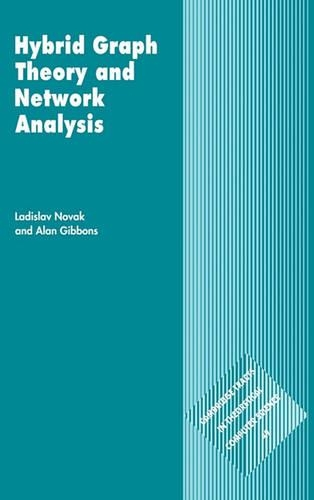•Cambridge Tracts in Theoretical Computer Science: Hybrid Graph Theory and Network Analysis Series Number 49 (Hardback)

(author), (author)
£82.00
Hardback 188 Pages / Published: 02/09/1999
• We can order this

Usually dispatched within 3 weeks

First published in 1999, this book combines traditional graph theory with the matroidal view of graphs and throws light on mathematical aspects of network analysis. This approach is called here hybrid graph theory. This is essentially a vertex-independent view of graphs naturally leading into the domain of graphoids, a generalisation of graphs. This enables the authors to combine the advantages of both the intuitive view from graph theory and the formal mathematical tools from the theory of matroids. A large proportion of the material is either new or is interpreted from a fresh viewpoint. Hybrid graph theory has particular relevance to electrical network analysis, which was one of the earliest areas of application of graph theory. It was essentially out of developments in this area that hybrid graph theory evolved.

Publisher: Cambridge University Press
ISBN: 9780521461177
Number of pages: 188
Weight: 450 g
Dimensions: 229 x 152 x 14 mm